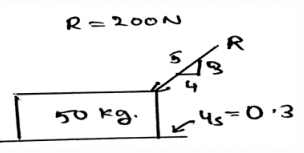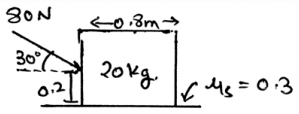# Test: Characteristics Of Dry Friction - 1

## 25 Questions MCQ Test Engineering Mechanics | Test: Characteristics Of Dry Friction - 1

Description
This mock test of Test: Characteristics Of Dry Friction - 1 for Mechanical Engineering helps you for every Mechanical Engineering entrance exam. This contains 25 Multiple Choice Questions for Mechanical Engineering Test: Characteristics Of Dry Friction - 1 (mcq) to study with solutions a complete question bank. The solved questions answers in this Test: Characteristics Of Dry Friction - 1 quiz give you a good mix of easy questions and tough questions. Mechanical Engineering students definitely take this Test: Characteristics Of Dry Friction - 1 exercise for a better result in the exam. You can find other Test: Characteristics Of Dry Friction - 1 extra questions, long questions & short questions for Mechanical Engineering on EduRev as well by searching above.
QUESTION: 1

### ____________ is the phenomena that resist the movement of the two surfaces in contact.

Solution:

The friction is the phenomena that defines that there is a resistance which is present there between the two surfaces. The two surfaces are in contact and the friction applies at that surface only, resisting the motion of the surface.

QUESTION: 2

### The frictional force always acts ____________ to the surface of the application of the friction.

Solution:

The friction is the phenomena that defines that there is a resistance which is present there between the two surfaces. This friction is applied tangentially to the surfaces in contact. Thus the main thing is that the forces on both of the surfaces act tangential to each other.

QUESTION: 3

### Dry friction is also called _____________

Solution:

The dry friction is acted upon the surfaces. And they are tangential to each other. As we know the friction is the phenomena that defines that there is a resistance which is present there between the two surfaces. The dry friction is also termed as the Coulomb friction as it was given by C.A. Coulomb.

QUESTION: 4

Who gave the concept of Dry friction?

Solution:

The dry friction is also termed as the Coulomb friction as it was given by C.A. Coulomb. And they are tangential to each other. As we know the friction is the phenomena that defines that there is a resistance which is present there between the two surfaces.

QUESTION: 5

At what conditions the dry friction occurs between the surfaces in contact?

Solution:

When there is no lubricating fluid present between the surfaces in contact, the dry friction occurs. Thus as the name suggests, dry friction. When there is no liquid present over there the surfaces are going to be said dry only. Thus the term dry friction.

QUESTION: 6

The phenomena of horizontal pull and push explains what?

Solution:

As we know that when there is no lubricating fluid present between the surfaces in contact, the dry friction occurs. This is the phenomena that defines that there is a resistance which is present there between the two surfaces. Thus the pull and push phenomena explains the theory of friction.

QUESTION: 7

The phenomena of horizontal pull and push explains what?

Solution:

As we know that when there is no lubricating fluid present between the surfaces in contact, the dry friction occurs. This is the phenomena that defines that there is a resistance which is present there between the two surfaces. Thus the pull and push phenomena explains the theory of friction.

QUESTION: 8

The block used to explain the theory of friction is kept over non-deformable surface.

Solution:

The block used to explain the theory of friction is a used in a deformable surface. As we know that the theory of friction says that when there is no lubricating fluid present between the surfaces in contact, the dry friction occurs. Thus to show the same experiments are done over a non-rigid/deformable surface.

QUESTION: 9

In the explanation of the theory of friction, the block used have an assumption. That is the upper portion of the block is considered to be rigid.

Solution:

The block used to explain the theory of friction is placed on a deformable surface. As we know that the theory of friction says that when there is no lubricating fluid present between the surfaces in contact, the dry friction occurs. Thus to show the same, experiments are done over a non-rigid/deformable surface which is having a rigid shape.

QUESTION: 10

The free body diagram used to explain the theory of dry friction is having ___________ distribution of both the normal forces and frictional surface.

Solution:

As we know that when there is no lubricating fluid present between the surfaces in contact, the dry friction occurs. This is the phenomena that defines that there is a resistance which is present there between the two surfaces. Thus the pull and push phenomena explains the theory of friction. Thus the free body diagrams are having uneven distribution of the forces.

QUESTION: 11

For equilibrium the normal forces acts in which direction in the free body diagrams?

Solution:

As the loads are being acting in the downward direction. Thus to make the forces balance, the normal forces act in the vertically upward direction. As we know that when there is no lubricating fluid present between the surfaces in contact, the dry friction occurs. This friction magnitude is taken out from these normal forces.

QUESTION: 12

What is not the condition for the equilibrium in free body diagram for calculation of the normal forces?

Solution:

For the equilibrium in the three dimensional system of axis we have all the conditions true as, ∑Fx=0, ∑Fy=0 and ∑Fz=0. Also we have the summation of the forces equal to zero. Which is not a non-zero value.

QUESTION: 13

We first make equilibrium equations of the body by considering all the three dimensional forces acting on the section chosen and then the free body diagram is made and solved.

Solution:

We first make the free body diagram and then we make the equilibrium equations to satisfy the given conditions. This helps us to solve the question easily. As this reduces the part of imagination and increases accuracy too.

QUESTION: 14

Calculate the product of normal force and the coefficient of friction between the body and the surface.Solution:

The net forces acting on the body is shown by the help of the resultant forces. There are two types, first the frictional and the second is the normal. This is because the resultant forces have the sum of all the forces which are acting on the direction which is same.

QUESTION: 15

Determine the distance between the points of action of the forces, that is the normal force and the self-weight force in the given figure.Solution:

The net forces acting on the body is shown by the help of the resultant forces. There are two types, first the frictional and the second is the normal. This is because the resultant forces have the sum of all the forces which are acting on the direction which is same.

QUESTION: 16

For making the equilibrium equations the normal forces acts in which direction in the free body diagrams?

Solution:

As the loads are being acting in the downward direction. Thus to make the forces balance, the normal forces act in the vertically upward direction. As we know that when there is no lubricating fluid present between the surfaces in contact, the dry friction occurs. This friction magnitude is taken out from these normal forces.

QUESTION: 17

Which one is not the condition for the equilibrium in free body diagram for calculation of the normal forces, consider all forces to be straight and linear?

Solution:

For the equilibrium in the three dimensional system of axis we have all the conditions true as, ∑Fx=0, ∑Fy=0 and ∑Fz=0. Also we have the summation of the forces equal to zero. Which is not a non-zero value.

QUESTION: 18

We first make equilibrium equations of the body by considering all the three dimensional forces acting on the section chosen and then the free body diagram is made and solved.

Solution:

We first make the free body diagram and then we make the equilibrium equations to satisfy the given conditions. This helps us to solve the question easily. As this reduces the part of imagination and increases accuracy too.

QUESTION: 19

We show the net forces by the help of __________ forces.

Solution:

The net forces acting on the body is shown by the help of the resultant forces. This is because the resultant forces have the sum of all the forces which are acting on the direction which is same. Thus the resultant forces are used to show the net forces acting in the body.

QUESTION: 20

There are main two types of forces which are being stated in the free body diagram, they are generally the resultant forces which are being acted over the body. Which are they?

Solution:

The net forces acting on the body is shown by the help of the resultant forces. There are two types, first the frictional and the second is the normal. This is because the resultant forces have the sum of all the forces which are acting on the direction which is same.

QUESTION: 21

In the problems regarding the friction and normal force calculations there is no scope of the two force and three force systems.

Solution:

The two and three force member system can be acted upon the body even if there is friction calculations involved. The main thing is that the frictional forces are the phenomena in which there is a resistance between the surfaces. And the resistance magnitude is given by those calculations. So if two or three system does comes, it has no effect.

QUESTION: 22

The difference between the two and the three force members is:

Solution:

The definition of the two force member only defines that the forces are being acted on the two points on the body. So does is the definition of the three forces members. The points of action of the three forces are three.

QUESTION: 23

There is the application of the Newton’s third law of motion in the free body diagrams of the friction calculations.

Solution:

There is some calculations involved in which we have to make use of the Newton’s third law of motion. The requirement of the third law is important in the equilibrium of the body. The body particles are in the equilibrium and are thus facing the forces and to be in the equilibrium they also react and apply the opposite force and thus the third law of newton.

QUESTION: 24

What does the Newton’s third law states?

Solution:

The requirement of the third law is important in the equilibrium of the body. The body particles are in the equilibrium and are thus facing the forces and to be in the equilibrium they also react and apply the opposite force and thus the third law of Newton. Thus there is some involvement of the Newton’s third law of motion.

QUESTION: 25

The net moment of the body is zero that means the distance between the force and the rotational axis is zero.

Solution:

The net moment of the body is zero that doesn’t means that the distance between the force and the rotational axis is zero. This thing is used when there is any rotational effect possible during the friction. For e.g. if we have a wedge and the block is placed over it then.